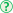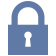Updated: 3/13/2018

# Resistance, Pressure, and Flow

0%
Topic
Review Topic
0
0
N/A
N/A
Questions
3
0
0
0%
0%
Evidence
4
0
0
Topic
 Vessel Pressure Overviewa change in pressure in a vessel is equal to flow times resistance:  ΔP = Q x R this is similar to Ohm's law where a change in voltage is equal to current times resistance:  ΔV = IR a pressure gradient drives flow from high pressure to low Resistance Formularesistance = (driving pressure ΔP) / (flow Q) = (8η)(viscosity)(length) / (πr4) is directly proportional to viscosity and inversely proportional to the radius of a vessel raised to the 4th powerPeripheral resistance arterioles account for most of the total peripheral resistance in the cardiovascular system regulates capillary flow Total resistance the total resistance of vessels in series is determined by summing the resistance of each vessel:  RTOTAL  = R1 + R2 + R3 + ... the total resistance of vessels in parallel is determined by taking the inverse of the sum of the inverses of the resistance of each vessel:  1/RTOTAL= 1/R1 + 1/R2 + 1/R3 + ... these calculations are done similarly to adding electrical resistors Viscosity Viscosity depends mostly on hematocrit Viscosity is increased in several disease states: polycythemia conversely, anemia decreases the viscosity hyperproteinemic states (e.g., multiple myeloma) hereditary spherocytosis

Average 4.3 of 8 Ratings

Questions (3)Sorry, this question is forSorry, this question is for

(M1.CV.12.79) A peripheral artery is found to have 50% stenosis. Therefore, compared to a normal artery with no stenosis, by what factor has the flow of blood been decreased?

QID: 100595
1

2

8%

(12/145)

2

4

16%

(23/145)

3

8

13%

(19/145)

4

16

59%

(85/145)

5

32

3%

(5/145)

M 1 E

Select Answer to see Preferred Response

Evidence (4)